# Review: Fast R-CNN (Object Detection)

In this story, Fast Region-based Convolutional Network method (Fast R-CNN)  is reviewed. It improves the training and testing speed as well as increasing the detection accuracy.

1. Fast R-CNN trains the very deep VGG-16  9× faster than R-CNN , 213× faster at test time
2. Higher mAP on PASCAL VOC 2012
3. Compared to SPPNet , it trains VGG-16 3× faster, tests 10× faster, and is more accurate.

This is an 2015 ICCV paper with over 3000 citations when I was writing this story. (Sik-Ho Tsang @ Medium)

### What are covered

1. The Problems of Prior Arts
2. ROI Pooling Layer
3. Multi-task Loss
4. Some Other Ablation Study
5. Comparison with State-of-the-art Results

### 1. The Problems of Prior Arts

#### 1.1. Multi-stage Pipeline

R-CNN and SPPNet first trains the CNN for softmax classifier, then uses the feature vectors for training the bounding box regressor. Thus, R-CNN and SPPNet are not end-to-end training.

#### 1.2. Expensive in Space and Time

As the feature vectors are stored in harddisk, occupied hundreds of gigabyte, for training the bounding box regressor.

#### 1.3. Slow Object Detection

At test-time, R-CNN using VGG-16 needs 47s per image using GPU which is slow.

Fast R-CNN solves above problems!

### 2. ROI Pooling Layer

This is actually a special case of SPP layer in SPPNet with only one pyramid used. Below illustrates the example: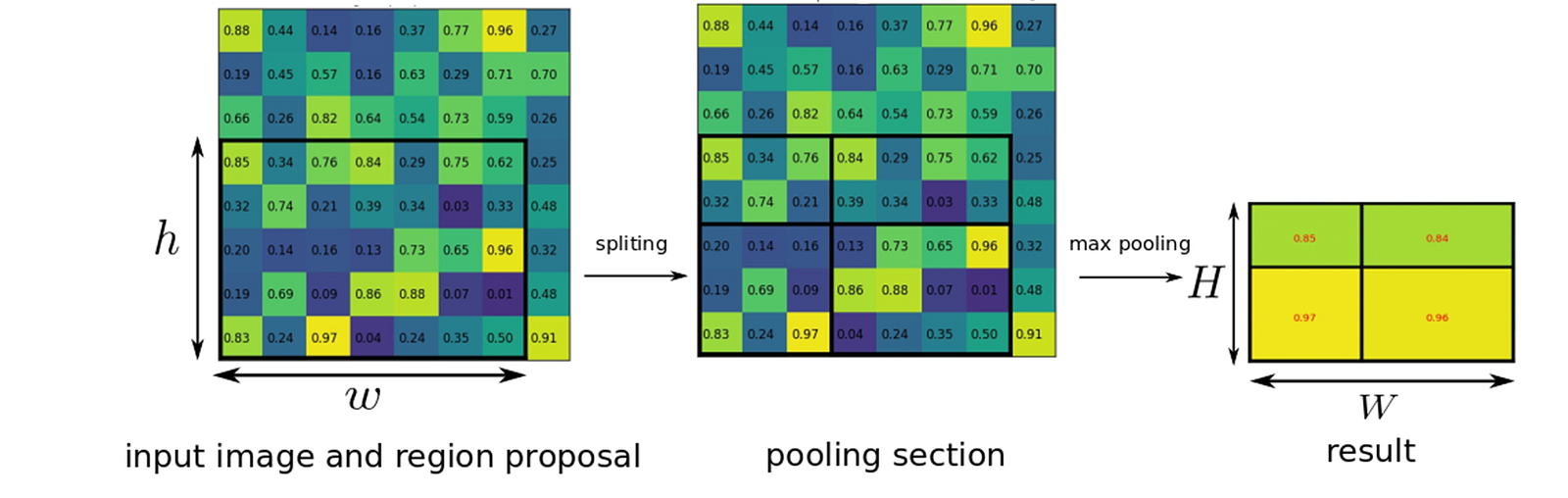ROI Pooling

Suppose we got the region proposal (left) with h×w, and we would like to have an output (right) of H×W sizes of output layer after pooling. Then, the area for each pooling area (middle) = h/H × w/W.

And in the example above, with input ROI of 5×7, and output of 2×2, the area for each pooling area is 2×3 or 3×3 after rounding.

And the maximum value within the pooling window is taken as output value for each grid which is the same idea of conventional max pooling layer.

### 3. Multi-task Loss

Since Fast R-CNN is an end-to-end learning architecture to learn the class of object as well as the associated bounding box position and size, the loss is multi-task loss.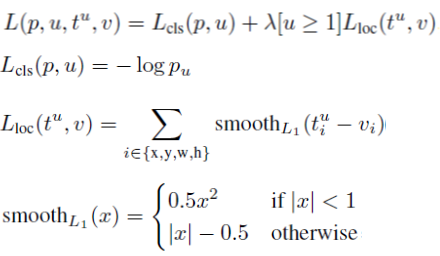Multi-task Loss

L_cls is the log loss for true class u.
L_los is the loss for bounding box.
[u≥1] means it is equal to 1 when u≥1. (u=0 is background class)

Compared with OverFeat, R-CNN, and SPPNet, Fast R-CNN uses multi-task loss to achieve end-to-end learning.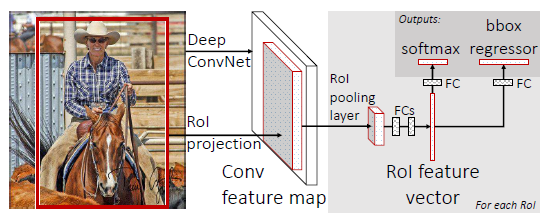Fast R-CNN

With mutli-task loss, at the ouput, we have softmax and bounding box regressor as shown at the top right of the figure.

3 Models are evaluated:
S = AlexNet or CaffeNet
M = VGG-like wider version of S
L = VGG-16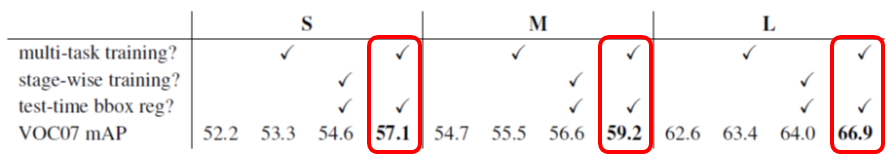Multi-task Loss Results

With multi-task loss, higher mAP is obtained compared with stage-wise training, i.e. separate training of softmax and bounding box regressor.

### 4. Some Other Ablation Study

#### 4.1 Multi Scale Training and Testing

An input image is tested using 5 scales.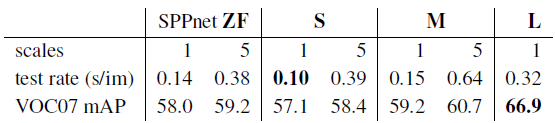1-Scale vs 5-Scale

With 5-scale, higher mAP is obtained for every model with the cost of larger test rate (seconds/image).

#### 4.2 SVM vs Softmax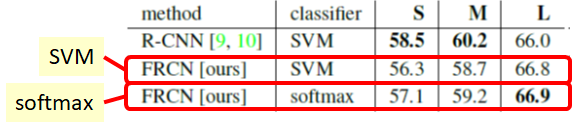SVM vs Softmax

In Fast R-CNN (FRCN), softmax is better than SVM.

Also, for SVM, the feature vectors need to be stored for hundreds of gigabyte in harddisk, and become stage-wise training while softmax can achieve end-to-end learning without storing feature vectors into harddisk.

#### 4.3 Region Proposals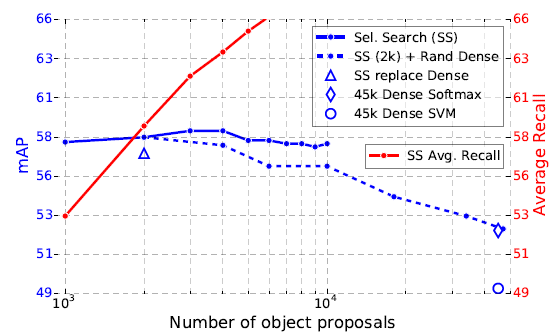Different Proposal Approaches

It is found that increasing number of region proposals does not necessary increase mAP.

Spare Set using Selective Search (SS)  is already good enough as shown in the figure above (Blue solid line) (SS  is being used in R-CNN.)

It is still a problem that Fast R-CNN needs region proposals from an external source.

#### 4.4 Truncated SVD for faster detection

One of the bottlenecks of testing time is at FC layers.

Authors use Singular Vector Decomposition (SVD) to reduce the number of connection in order to decrease the test time.

The top 1024 singular values from 25088×4096 matrix in FC6 layer, and the top 256 singular values from 4096×4096 matrix in FC7 layer.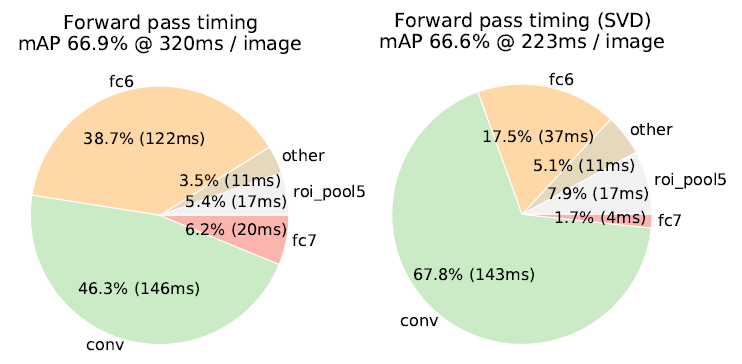Large Reduction of Test Time for FC6 and FC7 Layers

### 5. Comparison with State-of-the-art Results

#### 5.1 VOC 2007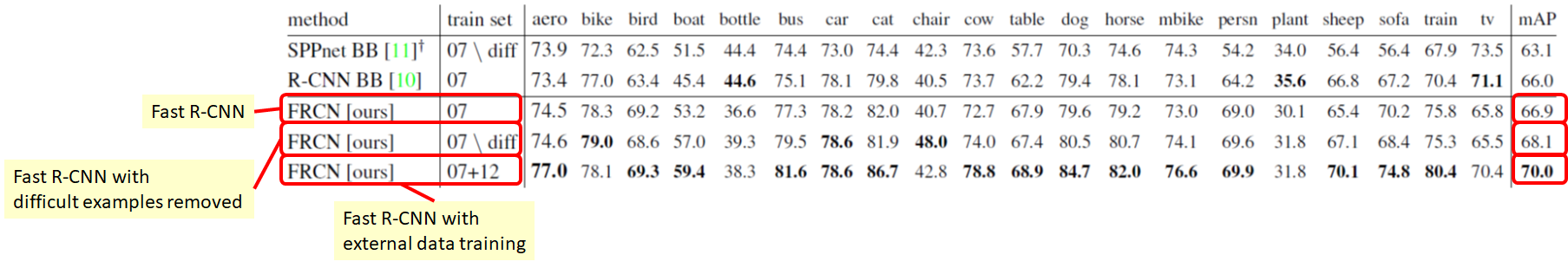VOC 2007 Results

Fast R-CNN: 66.9% mAP
Fast R-CNN with difficult examples removed during training (This is the setting of SPPNet): 68.1% mAP
Fast R-CNN with external VOC 2012 trained: 70.0% mAP

#### 5.2 VOC 2010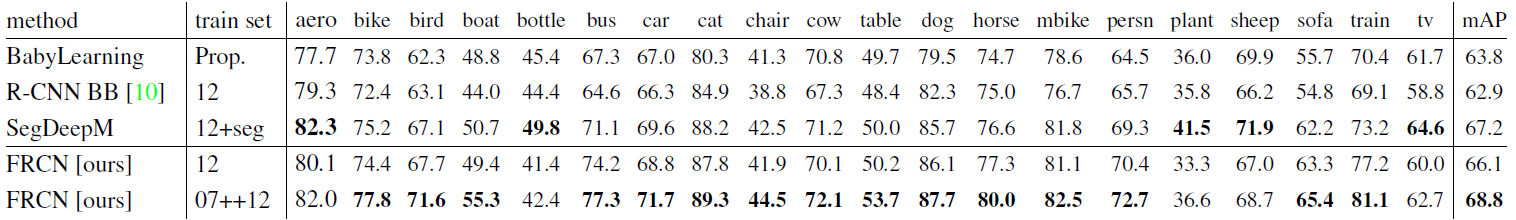VOC 2010 Results

Similar to VOC 2007, Fast R-CNN with external VOC 2007 and 2012 trained is the best with 68.8% mAP.

#### 5.3 VOC 2012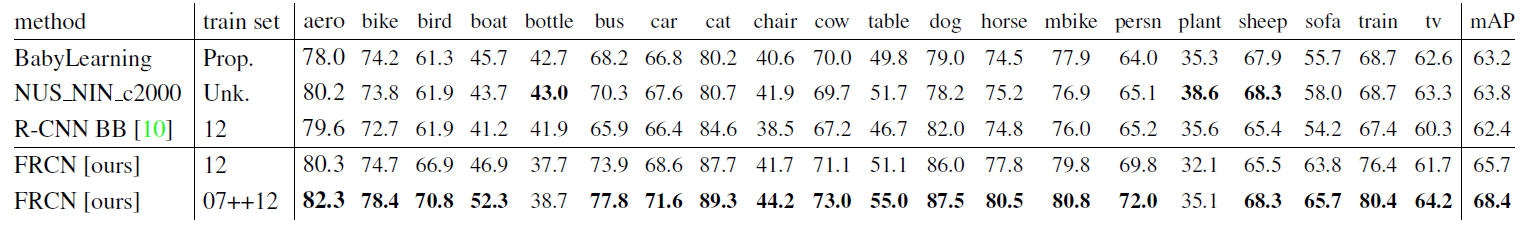VOC 2012 Results

Similar to VOC 2007, Fast R-CNN with external VOC 2007 trained is the best with 68.4% mAP.

#### 5.4 Training and Testing Time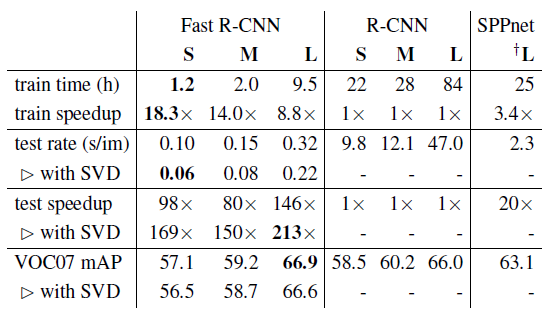Training and Testing Time

As mentioned, Fast R-CNN trains the very deep VGG-16  9× faster than R-CNN , 213× faster at test time.

Compared to SPPNet , it trains VGG-16 3× faster, and tests 10× faster.